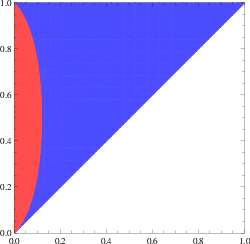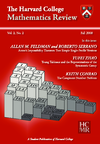# Research

My papers are listed below by topic.

## Research themes

• Graph regularity and additive combinatorics: Szemerédi’s graph regularity method is a powerful tool in modern graph theory research. The classical version of the regularity method only works in the dense setting, and my work extends the method to the sparse setting. Ideas arising from graph regularity can be transferred to the world of additive combinatorics. For example, our work on sparse graph/hypergraph regularity counting lemmas led to a simplification of the proof the Green–Tao theorem (see exposition).
• Regularity and counting lemmas in sparse graphs
• Arithmetic regularity, and applications to additive combinatorics
• Popular differences
• Property testing and algorithmic applications
• Graph limits
• Graph inequalities: Many fundamental problems in extremal graph theory can be phrased in terms of inequalities between subgraph densities or graph homomorphism counts. My work develops new tools to solve such problems.
• Independent sets, colorings, and graph homomorphisms
• Sidorenko’s conjecture and related problems
• Variational problems arising from large deviations in random graphs
• Discrete geometry: I am interested in extremal problems in discrete geometry, e.g., what is the largest size of a geometric configuration satisfying certain properties. Solutions often apply a variety of tools such as linear programming bounds, the polynomial method, spectral graph theory, group representation theory.
• Sphere packing, spherical codes
• Equiangular lines, spherical two-distance sets
• Incidence geometry, e.g., the joints problem
• The geometry of transitive sets, applications to Cayley graphs
• Extension complexity of polytopes

## Papers

### The Green-Tao theorem and a relative Szemerédi theorem slidesvideo### Sparse graph regularity and counting### Algorithmic graph regularity### Equiangular lines and eigenvalue multiplicities### Extension complexity and nonnegative rank### Plank problem### Independent sets and graph homomorphisms### Sphere packing and energy minimization### Large deviations in random graphs slides### Extremal subgraph density problems in directed graphs and tournaments### More sums than differences sets slides### Older expository papers and notes